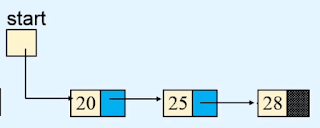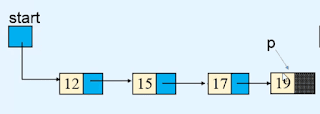today we are going to learn how to merge a linked list into another linked list. like we have two linked lists as you see in the images given below.

a second linked list has three nodes.so we want to merge the second list into the first list. so first we need to find the reference of the last node of the first linked list.and then we store the second linked list's first node's reference into the linked part of the last of the first linked list.

### Python program for merging one list into another linked list.

```class Node:

def __init__(self,value):
self.info = value

def __init__(self):
self.start = None
def display_list(self):
if self.start is None:
print("List is empty")
return        else:
print("List is : ")
p = self.start
while p is not None:
print(p.info, " ", end='')
print()

def insert_at_end(self, data):
temp = Node(data)
if self.start is None:
self.start = temp
return
p = self.start
while p.link is not None:

def create_list(self):
n = int(input("Enter the numer of nodes : "))
if n == 0:
return        for i in range(n):
data = int(input("Enter the element to be inserted : "))
self.insert_at_end(data)

def concatenate(self, list2):
if self.start is None:
self.start = list2.start
return
if list2.start is None:
return
p = self.start
while p.link is not None:

############################################################

print("Enter first list :- ")
list1.create_list()
print("Enter second list :- ")
list2.create_list()

print("First ")
list1.display_list()
print("Second ")
list2.display_list()

list1.concatenate(list2)
print("First ")
list1.display_list()```